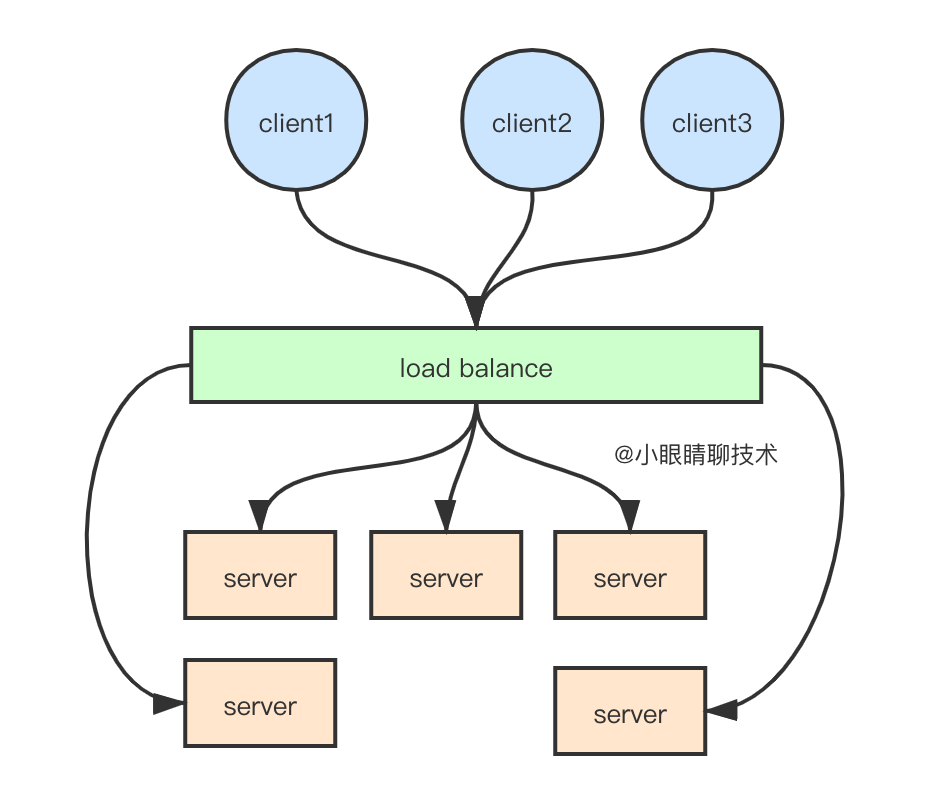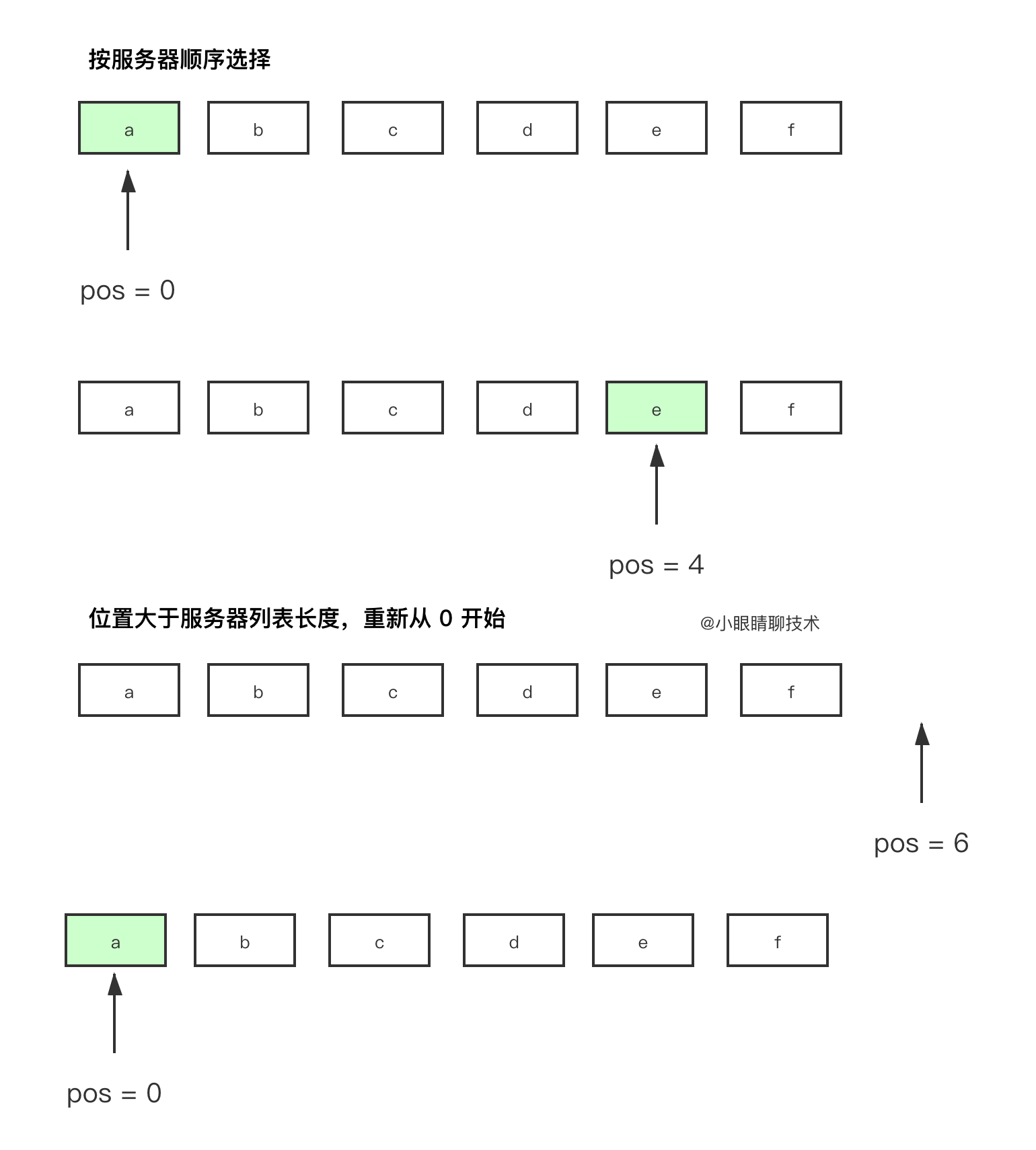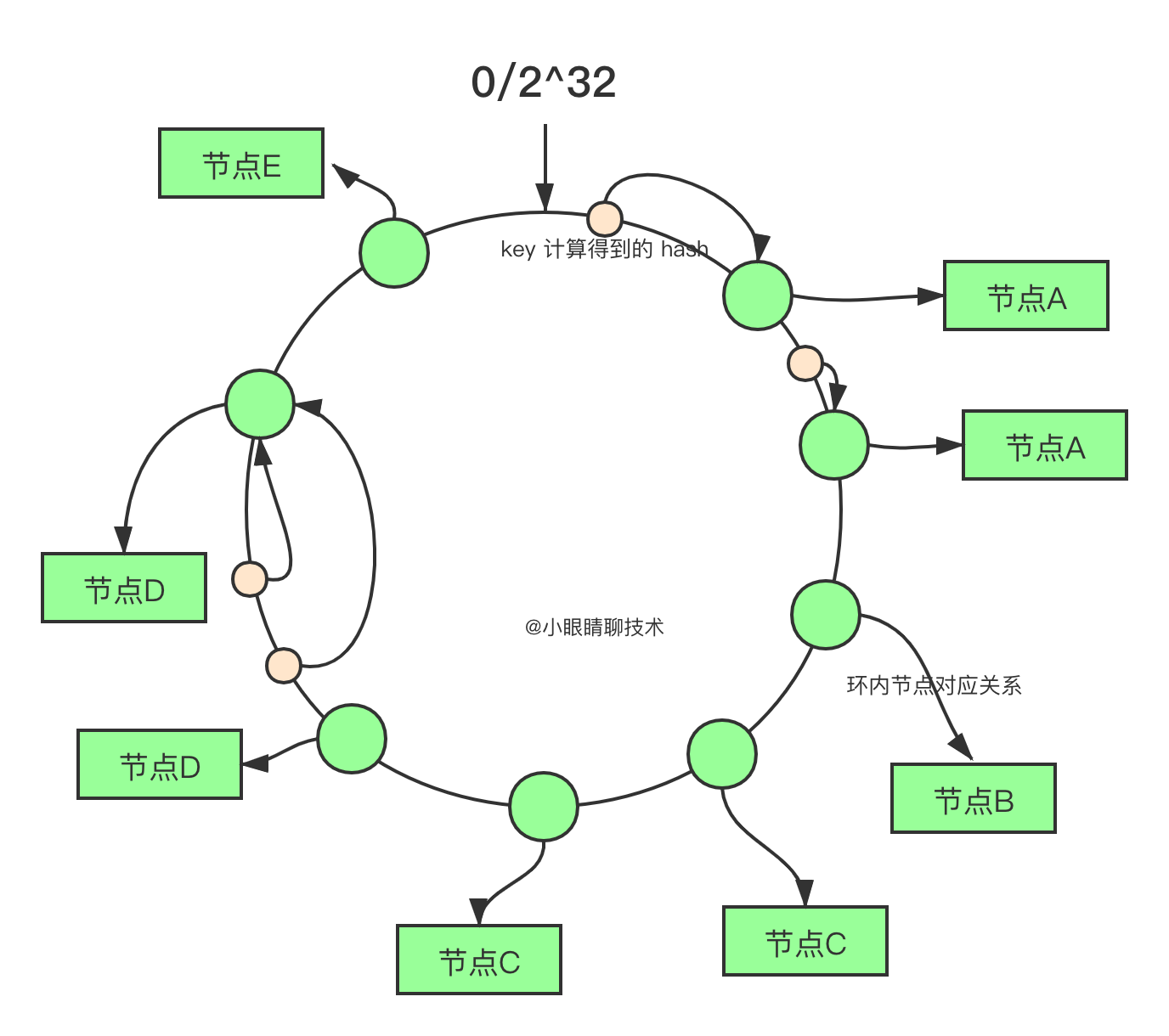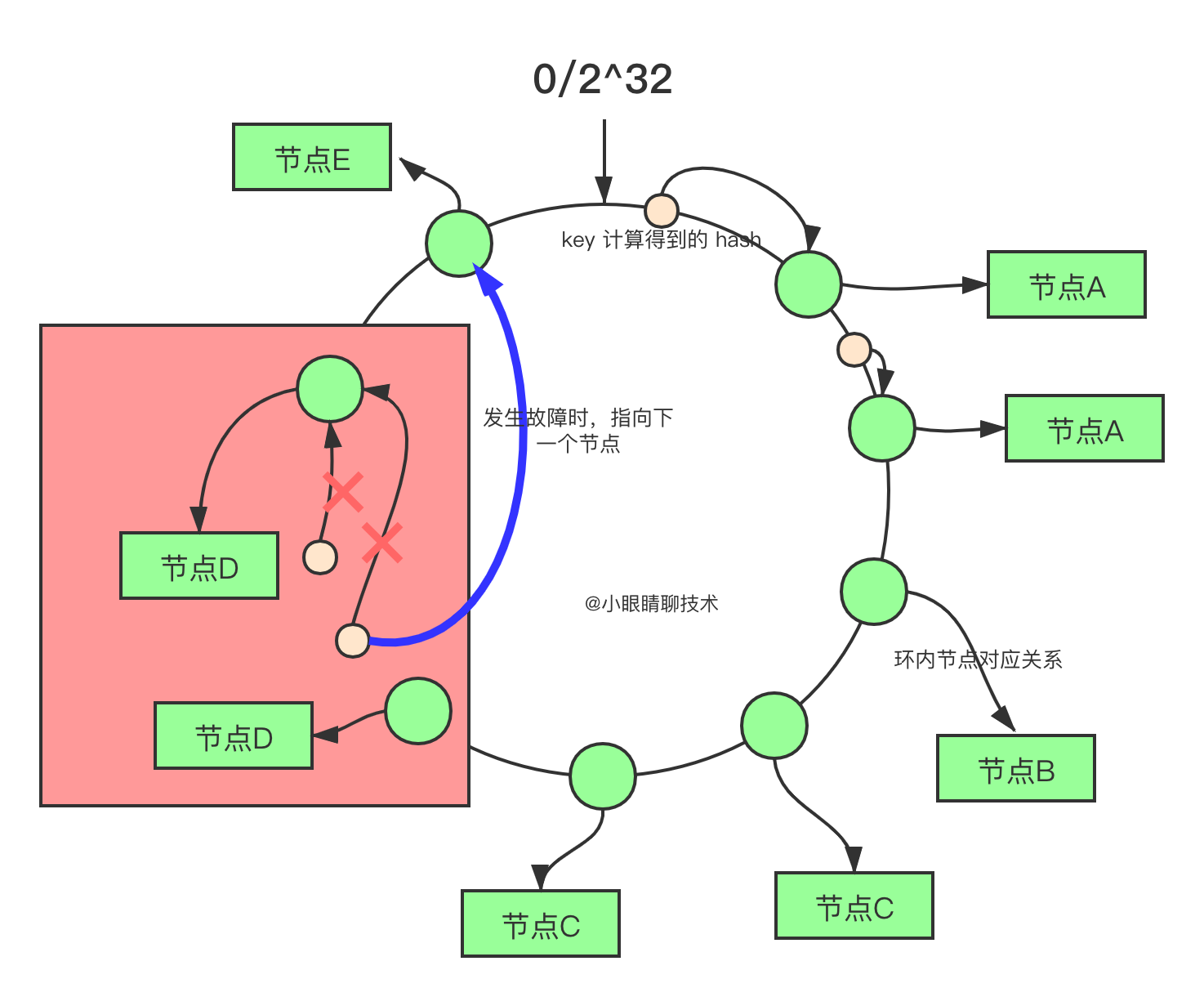# 手写负载均衡算法# 概述

﻿

「负载均衡」是指，通过一定的算法使请求可以均匀的宠幸服务提供方，做到雨露均沾。市面上，软件硬件产品一大把，解决的最最核心的问题都是选谁

﻿﻿

﻿

﻿

﻿

﻿

# 常见算法

﻿﻿

### 1. 随机（Random）

﻿

public Balanceable choice(Balanceable[] servers) {    int index = (int) (Math.random() * servers.length);    return servers[index];}

﻿

### 2. 权重随机（Weighted Random）

﻿

public Balanceable choice(Balanceable[] servers) {    int seed = 0;    for (Balanceable server : servers) {        seed += server.getWeight();    }    int random = r.nextInt(seed);    Collections.sort(Arrays.asList(servers));    int tmp = 0;    for (Balanceable server : servers) {        tmp += server.getWeight();        if (tmp >= random) {            return server;        }    }    return null;}

﻿﻿

﻿

• 所有权重相加得到 S（其实就是直线的长度）

• 从 [0, S) 的区间内取一个随机数 R（直线中随机选择一个点）

• 遍历节点列表，把访问过的节点的权重相加得到 V，比较 V 与 R 的值，如果 V > R 当前节点即为选中的节点。（查找 R 在直线中的位置属于哪个节点所在的区域）

﻿

### 3. 轮询（Round Robin）

﻿

﻿

Integer pos = 0;public Balanceable choice(Balanceable[] servers) {    Balanceable result = null;    synchronized(pos) {        if (pos >= servers.length){            pos = 0;        }        result = servers[pos];        pos++;    }    return result;}

﻿﻿

### 4. 最小连接数（Least Connections）

﻿

﻿

public Balanceable choice(Balanceable[] servers) {    int length = servers.size();                                                                                          int leastActive = -1;    int leastCount = 0;     int[] leastIndexs = new int[length];     int totalWeight = 0;     int firstWeight = 0;     boolean sameWeight = true;     for (int i = 0; i < length; i++) {                                                                                                                                     Balanceable invoker = servers[i];        int active = status.getStatus(servers).getActive();         int weight = server.getWeight();                 if (leastActive == -1 || active < leastActive) {             leastActive = active;             leastCount = 1;             leastIndexs = i;             totalWeight = weight;             firstWeight = weight;             sameWeight = true;         } else if (active == leastActive) {             leastIndexs[leastCount++] = i;             totalWeight += weight;             if (sameWeight && i > 0                    && weight != firstWeight) {                sameWeight = false;            }        }    }        if (leastCount == 1) {             return servers[leastIndexs];    }    if (!sameWeight && totalWeight > 0) {            int offsetWeight = random.nextInt(totalWeight);        for (int i = 0; i < leastCount; i++) {            int leastIndex = leastIndexs[i];            offsetWeight -= getWeight(servers[leastIndex]);            if (offsetWeight <= 0)                return servers[leastIndex];        }    }        return servers[leastIndexs[random.nextInt(leastCount)]];}

﻿

﻿

### 5. 一致性哈希（Consistent Hash）

﻿

﻿﻿

﻿﻿

• 构造哈希环

﻿

public static TreeMap<Long, String> populateConsistentBuckets(			String... servers) {    // store buckets in tree map    TreeMap<Long, String> consistentBuckets = new TreeMap<Long, String>();    MessageDigest md5 = MD5.get();    int totalWeight = servers.length;    for (int i = 0; i < servers.length; i++) {        int thisWeight = 1;        double factor = Math            .floor(((double) (40 * servers.length * thisWeight))                   / (double) totalWeight);        for (long j = 0; j < factor; j++) {            byte[] d = md5.digest((servers[i] + "-" + j).getBytes());            for (int h = 0; h < 4; h++) {                Long k = ((long) (d[3 + h * 4] & 0xFF) << 24)                    | ((long) (d[2 + h * 4] & 0xFF) << 16)                    | ((long) (d[1 + h * 4] & 0xFF) << 8)                    | ((long) (d[0 + h * 4] & 0xFF));                consistentBuckets.put(k, servers[i]);            }        }    }    return consistentBuckets;}

﻿

• 从环中找到合适的节点：

﻿

public static final Long getBucket(TreeMap<Long, String> buckets, Long hv) {    SortedMap<Long, String> t = buckets.tailMap(hv);    return (t.isEmpty()) ? buckets.firstKey() : t.firstKey();}

﻿

# 总结

﻿

1. 负载均衡，目的是让每台服务器获取到适合自己处理能力的负载

2. 可以采用硬件、软件的方式进行实现

3. 常见的几种负载均衡算法，各自有优缺点，选择不同场景使用

﻿﻿

# 关于我

﻿﻿## 评论